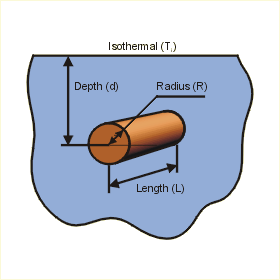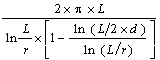Close

This page calculates the temperature (Tc ) of an isothermal (constant temperature) cylinder embedded in a semi-infinite medium at a specified depth (d) from an isothermal surface.The cylinder temperature Tc is calculated as:

Tc = Ti + q / (k x sf)

Where Ti is the isothermal temperature applied to the medium surrounding the cylinder, q is the heat flow, sf is the shape factor, and k is the conductivity of the medium.

The shape factor depends on the relationships between  the length and the radius, depth and the radius, as well as the length and the radius. Three cases can be calculated.

Case 1:

When the cylinder length is much larger than the cylinder radius (at least 10 times greater) and the depth is greater than or equal to the length of the cylinder, then the following equation is used:

sf = 2 pi x L / (cosh-1 ( d / r) )

Case 2:

When the cylinder length is much larger than the cylinder radius (at least 10 times larger) and the depth is greater than the three times cylinder radius, then another equation can be applied:

sf = 2 pi x L / ( ln (2 d / r) )

Case 3:

When the depth of the embedded cylinder is much larger than the cylinder radius (at least 10 times larger) and the cylinder length is much larger than the depth of the embedded cylinder (at least 10 times larger), the shape factor is calculated as:

sf =Where r is the cylinder radius, L is the cylinder length, and d is the cylinder depth.

References

Holman, J.P., Heat Transfer, 7th ed., McGraw Hill Book Company, New York, 1990. p 74 - 83